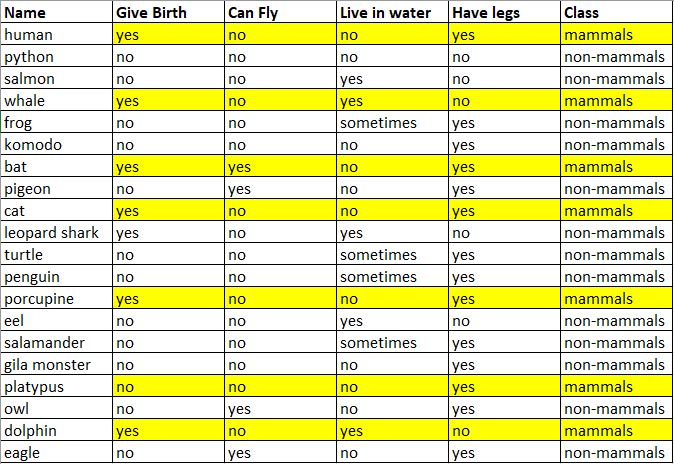## Jorunal 4 Intelligent Systems: Naive Bayes

by jerdy on 23/11/2019

Naive Bayes classifiers are a collection of classification algorithms based on Bayes’ Theorem. Naive Bayes classifier is a family of algorithms where all of the algorithms share a common principle. Every classified feature is independent of each other.

Bayes’ Theorem is a mathematical formula created by Thomas Bayes. Bayes’ Theorem is a mathematical formula for determining conditional probability. The theorem provides a way to revise existing predictions or theories (update probabilities) given new or additional evidence.

Now let us practiceGuess what the class of the given characteristics below:Give birth = yes, can fly = no, live in water = yes, have legs = no

The first step is to divide the classes into mammals and non-mammals. Then, we determine how many animals in each class.

The Mammals Class

The Non-mammals Class

The second step is to count the prior probability.

In total, there are 7 mammals and 13 non-mammals.

Divide 7 by 20 to get the prior probability of mammals.

Divide 13 by 20 to get the prior probability of non-mammals.

The third step is to count the probability.

Now, let us test the model if the instance is:

Give birth = yes, can fly = no, live in water = yes, have legs = no

Based on the equation:

P(mammals|give birth, can fly, live in water, have legs) = 0.020991254

P(non-mammals|give birth, can fly, live in water, have legs) = 0.002730997

The class that has the highest probability is: Mammals.

jerdy@binus.ac.id